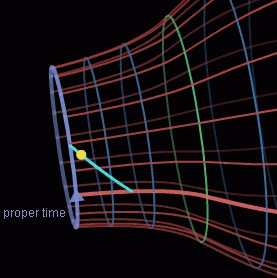Search IntMath
Close

# Curved spacetime simulation

By Murray Bourne, 30 Jan 2008

Adamtoons.de has some mind-blowing animations on gravitation as curved spacetime and relativity: movement, time dilation and length compression.There's also a charged ball simulation where you can create balls and observe their behavior under the influence of magnetic fields.

It's worth a look, especially if you are struggling with physics concepts.

### Comment Preview

HTML: You can use simple tags like <b>, <a href="...">, etc.

To enter math, you can can either:

1. Use simple calculator-like input in the following format (surround your math in backticks, or qq on tablet or phone):
a^2 = sqrt(b^2 + c^2)
(See more on ASCIIMath syntax); or
2. Use simple LaTeX in the following format. Surround your math with $$ and $$.
$$\int g dx = \sqrt{\frac{a}{b}}$$
(This is standard simple LaTeX.)

NOTE: You can mix both types of math entry in your comment.